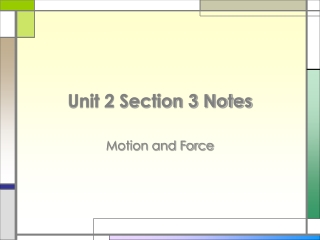DownloadDownload PresentationUnit 2 Section 3 Notes

Unit 2 Section 3 Notes

Télécharger la présentationUnit 2 Section 3 Notes

- - - - - - - - - - - - - - - - - - - - - - - - - - - E N D - - - - - - - - - - - - - - - - - - - - - - - - - - -
Presentation Transcript

1. Unit 2 Section 3 Notes Motion and Force

2. What is Force? • A force is the cause of acceleration or change in an object’s velocity

3. Fundamental Forces • There are 4 fundamental forces in nature: • Gravity • Electromagnetic force • Strong nuclear force • Weak nuclear force

4. Fundamental Forces • Gravity: very, very weak force; this force acts over a long distance; much weaker than the electromagnetic force; field force • Electromagnetic force: moderate force; acts over long distances; about 1/100 the strength of the strong force; field force

5. Fundamental Forces • Strong nuclear force: strongest of all forces, but acts over a very short distance; only acts over distances the size of the atomic nucleus • Holds together protons and neutrons in the nuclei of atoms • Weak nuclear force: associated with nuclear decay

6. Fundamental Forces

7. Types of Forces • Contact forces:caused when objects touch • Examples: when you push a shopping cart or catch a baseball and it stops moving • Field forces:do not require that objects touch each other • Examples: attraction of gravity or repulsion between 2 north poles of a magnet • Both contact and field forces can cause an object to move or stop moving!

8. Balanced and Unbalanced Forces • Net force: the sum of all the forces acting on an object • Whenever there is a net force acting on an object, the object accelerates in the direction of the net force.

9. Balanced and Unbalanced Forces • An object will not accelerate if the net force is equal to zero. • Balanced forces: net force of zero • Do not cause a change in motion of an object.

10. Balanced and Unbalanced Forces • Example of Balanced Forces: • When 2 teams are playing tug of war and both teams are exerting equal amounts of pressure, there is no acceleration, and neither team moves.

11. Balanced and Unbalanced Forces • Unbalanced forces: occurs when forces acting on an object don’t cancel out; there is a greater force acting on 1 side of the object • Example: When 2 teams are playing tug of war and one team exerts a greater force and pulls the other team forward

12. Balanced and Unbalanced Forces • When an object is at rest, are there still forces acting on it? • Yes! It is just not moving because the forces are balanced. • What happens if forces act in different directions that are not opposite one another? • The combination of forces acts like a single force and cause acceleration in a combined direction • Example: You push your book east and your friend pushes the book north; the book will move northeast

13. Friction • Friction: force between 2 objects that opposes the motion of either object • Example: Imagine a car rolling on a flat, evenly paved street. Eventually, the car will stop due to the force of friction. • Friction depends on surfaces in contact: • Frictional forces are greater when both surfaces are rough • Example: new tennis shoes walking along a carpeted floor will keep you from falling down.

14. Friction • Two types of friction: • Static Friction: when 2 objects touch but do not move • Example: A filing cabinet sitting on the floor

15. Friction • Kinetic Friction: friction between moving surfaces. Two types of kinetic friction: • Sliding friction: when 1 object slides over another • Example: Sliding a box across the counter • Rolling friction: when 1 object rolls across another • Example: Rollerblading

16. Friction • The force necessary to make a stationary object start moving is usually more than the force necessary to keep it moving; therefore, static friction is usually greater than kinetic friction. • Rolling friction is usually less than sliding friction.

17. Friction and Motion • Friction is not all bad; it is actually required for many everyday tasks to work correctly. • Examples: • Cars could not move without friction; as a car’s wheels turn, they push against the road and as a reaction, the road pushes forward on the car. • You would not be able to write down these notes either!

18. Friction and Acceleration

19. Air Resistance • Air Resistance: a type of friction • Air Resistance is caused by the interaction between the surface of a moving object and the air molecules • Air Resistance depends on 3 factors: • Size of the object • Shape of the object • Speed of the object

20. Gravity • Gravity: force of attraction between 2 particles of matter due to their mass • Gravity is different from other forces because: gravity acts on an object even when the objects don’t touch • All objects in the universe attract each other through the force of gravity!

21. Gravity depends on two factors: The mass of the objects: the greater the mass of the object, the larger the gravitational force The distance between the objects: as the distance between 2 objects increases, the gravitational force decreases. Gravity